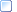﻿ Analog Data - Floating-point format
 The 3D Point Data Section > Analog Data Storage

### Analog Data - Floating-point format

When storing analog data using floating-point format, the analog information is stored as a floating-point value.  This should usually be the (12 to 16 bit resolution) analog sample value after conversion to a floating-point value – for example in the ADC sample value is 1024 then it must be stored as 1024.000 as a floating-point value.  Floating-point analog data storage is organized in exactly the same way, within the C3D file data section, as the integer analog data.  The stored analog data values must never be stored as pre-scaled values as this effectively destroys vital information about the analog data sampling and processing.

The parameters ANALOG:GEN_SCALE and channel specific ANALOG:SCALE and ANALOG:OFFSET values must be applied to the floating-point value to obtain physical world units in exactly the same way as we scale the integer formatted data.

Thus, a floating-point analog sample is calculated as:

physical world value = (data value - offset) * channel scale * general scale

Where:

‘offset’ is in the “ANALOG:OFFSET” parameters (integer)

‘channel scale’ is in the “ANALOG:SCALE” parameters (floating-point)

‘general scale’ is the “ANALOG:GEN_SCALE” parameter (floating-point)

More:Notes for programmers - Floating-point Analog Data